libMesh::DenseSubVector< T > Class Template Reference

#include <dense_subvector.h>

Inheritance diagram for libMesh::DenseSubVector< T >: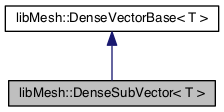[legend]

## Public Member Functions

DenseSubVector (DenseVector< T > &new_parent, const unsigned int ioff=0, const unsigned int n=0)

DenseSubVector (DenseSubVector &&)=default

DenseSubVector (const DenseSubVector &)=default

DenseSubVectoroperator= (const DenseSubVector &)=default

DenseSubVectoroperator= (DenseSubVector &&)=default

virtual ~DenseSubVector ()=default

DenseVector< T > & parent ()

virtual void zero () override

const T & operator() (const unsigned int i) const

T & operator() (const unsigned int i)

virtual T el (const unsigned int i) const override

virtual T & el (const unsigned int i) override

virtual unsigned int size () const override

virtual bool empty () const override

unsigned int i_off () const

void reposition (const unsigned int ioff, const unsigned int n)

Real min () const

Real max () const

Real l1_norm () const

Real l2_norm () const

Real linfty_norm () const

void print (std::ostream &os) const

void print_scientific (std::ostream &os, unsigned precision=8) const

## Private Attributes

DenseVector< T > & _parent_vector

unsigned int _n

unsigned int _i_off

## Detailed Description

### template<typename T> class libMesh::DenseSubVector< T >

Defines a dense subvector for use in finite element computations. Useful for storing element load vectors before summation into a global vector, particularly when you have systems of equations. All overridden virtual functions are documented in dense_vector_base.h.

Date
2003

Definition at line 42 of file dense_subvector.h.

## ◆ DenseSubVector() [1/3]

template<typename T >
 libMesh::DenseSubVector< T >::DenseSubVector ( DenseVector< T > & new_parent, const unsigned int ioff = 0, const unsigned int n = 0 )
inline

Constructor. Creates a dense subvector of the vector parent. The subvector has dimensions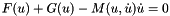, and the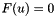entry of the subvector is located at the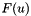location in the parent vector.

Definition at line 161 of file dense_subvector.h.

References libMesh::DenseSubVector< T >::reposition().

163  :
164  _parent_vector(new_parent)
165 {
166  reposition (ioff, n);
167 }
void reposition(const unsigned int ioff, const unsigned int n)
DenseVector< T > & _parent_vector

## ◆ DenseSubVector() [2/3]

template<typename T>
 libMesh::DenseSubVector< T >::DenseSubVector ( DenseSubVector< T > && )
default

The 5 special functions can be defaulted for this class, as it does not manage any memory itself.

## ◆ DenseSubVector() [3/3]

template<typename T>
 libMesh::DenseSubVector< T >::DenseSubVector ( const DenseSubVector< T > & )
default

## ◆ ~DenseSubVector()

template<typename T>
 virtual libMesh::DenseSubVector< T >::~DenseSubVector ( )
virtualdefault

## ◆ el() [1/2]

template<typename T>
 virtual T libMesh::DenseSubVector< T >::el ( const unsigned int i ) const
inlineoverridevirtual
Returns
The (i) element of the vector.

Implements libMesh::DenseVectorBase< T >.

Definition at line 83 of file dense_subvector.h.

84  { return (*this)(i); }

## ◆ el() [2/2]

template<typename T>
 virtual T& libMesh::DenseSubVector< T >::el ( const unsigned int i )
inlineoverridevirtual
Returns
The (i) element of the vector as a writable reference.

Implements libMesh::DenseVectorBase< T >.

Definition at line 86 of file dense_subvector.h.

87  { return (*this)(i); }

## ◆ empty()

template<typename T>
 virtual bool libMesh::DenseSubVector< T >::empty ( ) const
inlineoverridevirtual
Returns
true iff size() is 0.

Reimplemented from libMesh::DenseVectorBase< T >.

Definition at line 92 of file dense_subvector.h.

References libMesh::DenseSubVector< T >::_n.

Referenced by libMesh::NumericVector< Number >::insert().

93  { return (_n == 0); }

## ◆ i_off()

template<typename T>
 unsigned int libMesh::DenseSubVector< T >::i_off ( ) const
inline
Returns
The row offset into the parent vector.

Definition at line 98 of file dense_subvector.h.

References libMesh::DenseSubVector< T >::_i_off.

98 { return _i_off; }

## ◆ l1_norm()

template<typename T >
 Real libMesh::DenseSubVector< T >::l1_norm ( ) const
inline
Returns
The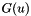-norm of the vector, i.e. the sum of the absolute values of the entries.

Definition at line 252 of file dense_subvector.h.

References std::abs(), and libMesh::Real.

253 {
254  Real my_norm = 0.;
255  for (unsigned int i=0; i!=this->size(); i++)
256  {
257  my_norm += std::abs(_parent_vector (i + this->i_off()));
258  }
259  return my_norm;
260 }
double abs(double a)
unsigned int i_off() const
virtual unsigned int size() const override
DenseVector< T > & _parent_vector
DIE A HORRIBLE DEATH HERE typedef LIBMESH_DEFAULT_SCALAR_TYPE Real

## ◆ l2_norm()

template<typename T >
 Real libMesh::DenseSubVector< T >::l2_norm ( ) const
inline
Returns
The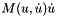-norm of the vector, i.e. the square root of the sum of the squares of the entries.

Definition at line 266 of file dense_subvector.h.

References libMesh::TensorTools::norm_sq(), and libMesh::Real.

267 {
268  Real my_norm = 0.;
269  for (unsigned int i=0; i!=this->size(); i++)
270  {
271  my_norm += TensorTools::norm_sq(_parent_vector (i + this->i_off()));
272  }
273  return sqrt(my_norm);
274 }
unsigned int i_off() const
virtual unsigned int size() const override
DenseVector< T > & _parent_vector
DIE A HORRIBLE DEATH HERE typedef LIBMESH_DEFAULT_SCALAR_TYPE Real

## ◆ linfty_norm()

template<typename T >
 Real libMesh::DenseSubVector< T >::linfty_norm ( ) const
inline
Returns
The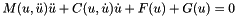-norm of the vector, i.e. the maximum absolute value of the entries.

Definition at line 280 of file dense_subvector.h.

References libMesh::TensorTools::norm_sq(), and libMesh::Real.

281 {
282  if (!this->size())
283  return 0.;
284  Real my_norm = TensorTools::norm_sq(_parent_vector (this->i_off()));
285
286  for (unsigned int i=1; i!=this->size(); i++)
287  {
288  Real current = TensorTools::norm_sq(_parent_vector (i + this->i_off()));
289  my_norm = (my_norm > current? my_norm : current);
290  }
291  return sqrt(my_norm);
292 }
unsigned int i_off() const
virtual unsigned int size() const override
DenseVector< T > & _parent_vector
DIE A HORRIBLE DEATH HERE typedef LIBMESH_DEFAULT_SCALAR_TYPE Real

## ◆ max()

template<typename T >
 Real libMesh::DenseSubVector< T >::max ( ) const
inline
Returns
The maximum element in the vector, or the maximum real part in the case of complex numbers.

Definition at line 235 of file dense_subvector.h.

References libMesh::libmesh_real(), and libMesh::Real.

236 {
237  libmesh_assert (this->size());
238  Real my_max = libmesh_real(_parent_vector (this->i_off()));
239
240  for (unsigned int i=1; i!=this->size(); i++)
241  {
242  Real current = libmesh_real(_parent_vector (i + this->i_off()));
243  my_max = (my_max > current? my_max : current);
244  }
245  return my_max;
246 }
T libmesh_real(T a)
unsigned int i_off() const
virtual unsigned int size() const override
DenseVector< T > & _parent_vector
DIE A HORRIBLE DEATH HERE typedef LIBMESH_DEFAULT_SCALAR_TYPE Real

## ◆ min()

template<typename T >
 Real libMesh::DenseSubVector< T >::min ( ) const
inline
Returns
The minimum element in the vector, or the minimum real part in the case of complex numbers.

Definition at line 218 of file dense_subvector.h.

References libMesh::libmesh_real(), and libMesh::Real.

219 {
220  libmesh_assert (this->size());
221  Real my_min = libmesh_real(_parent_vector (this->i_off()));
222
223  for (unsigned int i=1; i!=this->size(); i++)
224  {
225  Real current = libmesh_real(_parent_vector (i + this->i_off()));
226  my_min = (my_min < current? my_min : current);
227  }
228  return my_min;
229 }
T libmesh_real(T a)
unsigned int i_off() const
virtual unsigned int size() const override
DenseVector< T > & _parent_vector
DIE A HORRIBLE DEATH HERE typedef LIBMESH_DEFAULT_SCALAR_TYPE Real

## ◆ operator()() [1/2]

template<typename T >
 const T & libMesh::DenseSubVector< T >::operator() ( const unsigned int i ) const
inline
Returns
The (i,j) element of the subvector as a const reference.

Definition at line 197 of file dense_subvector.h.

198 {
199  libmesh_assert_less (i, this->size());
200  libmesh_assert_less (i + this->i_off(), _parent_vector.size());
201
202  return _parent_vector (i + this->i_off());
203 }
unsigned int i_off() const
virtual unsigned int size() const override
DenseVector< T > & _parent_vector

## ◆ operator()() [2/2]

template<typename T >
 T & libMesh::DenseSubVector< T >::operator() ( const unsigned int i )
inline
Returns
The (i,j) element of the subvector as a writable reference.

Definition at line 208 of file dense_subvector.h.

209 {
210  libmesh_assert_less (i, this->size());
211  libmesh_assert_less (i + this->i_off(), _parent_vector.size());
212
213  return _parent_vector (i + this->i_off());
214 }
unsigned int i_off() const
virtual unsigned int size() const override
DenseVector< T > & _parent_vector

## ◆ operator=() [1/2]

template<typename T>
 DenseSubVector& libMesh::DenseSubVector< T >::operator= ( const DenseSubVector< T > & )
default

## ◆ operator=() [2/2]

template<typename T>
 DenseSubVector& libMesh::DenseSubVector< T >::operator= ( DenseSubVector< T > && )
default

## ◆ parent()

template<typename T>
 DenseVector& libMesh::DenseSubVector< T >::parent ( )
inline
Returns
A reference to the parent vector.

Definition at line 68 of file dense_subvector.h.

References libMesh::DenseSubVector< T >::_parent_vector.

Referenced by libMesh::DenseSubMatrix< T >::condense().

68 { return _parent_vector; }
DenseVector< T > & _parent_vector

## ◆ print()

template<typename T >
 void libMesh::DenseVectorBase< T >::print ( std::ostream & os ) const
inherited

Pretty-print the vector to stdout.

Definition at line 51 of file dense_vector_base.C.

52 {
53  for (auto i : IntRange<int>(0, this->size()))
54  os << std::setw(8)
55  << this->el(i)
56  << std::endl;
57 }
virtual unsigned int size() const =0
virtual T el(const unsigned int i) const =0

## ◆ print_scientific()

template<typename T >
 void libMesh::DenseVectorBase< T >::print_scientific ( std::ostream & os, unsigned precision = 8 ) const
inherited

Prints the entries of the vector with additional decimal places in scientific notation.

Definition at line 31 of file dense_vector_base.C.

32 {
33  // save the initial format flags
34  std::ios_base::fmtflags os_flags = os.flags();
35
36  // Print the vector entries.
37  for (auto i : IntRange<int>(0, this->size()))
38  os << std::setw(10)
39  << std::scientific
40  << std::setprecision(precision)
41  << this->el(i)
42  << std::endl;
43
44  // reset the original format flags
45  os.flags(os_flags);
46 }
virtual unsigned int size() const =0
virtual T el(const unsigned int i) const =0

## ◆ reposition()

template<typename T >
 void libMesh::DenseSubVector< T >::reposition ( const unsigned int ioff, const unsigned int n )
inline

Changes the location of the subvector in the parent vector.

Definition at line 173 of file dense_subvector.h.

Referenced by libMesh::DenseSubVector< T >::DenseSubVector().

175 {
176  _i_off = ioff;
177  _n = n;
178
179  // Make sure we still fit in the parent vector.
180  libmesh_assert_less_equal ((this->i_off() + this->size()), _parent_vector.size());
181 }
unsigned int i_off() const
virtual unsigned int size() const override
DenseVector< T > & _parent_vector

## ◆ size()

template<typename T>
 virtual unsigned int libMesh::DenseSubVector< T >::size ( ) const
inlineoverridevirtual
Returns
The size of the vector.

Implements libMesh::DenseVectorBase< T >.

Definition at line 89 of file dense_subvector.h.

References libMesh::DenseSubVector< T >::_n.

Referenced by libMesh::NumericVector< Number >::insert().

90  { return _n; }

## ◆ zero()

template<typename T >
 void libMesh::DenseSubVector< T >::zero ( )
inlineoverridevirtual

Set every element in the vector to 0. Needs to be pure virtual since the storage method may be different in derived classes.

Implements libMesh::DenseVectorBase< T >.

Definition at line 187 of file dense_subvector.h.

188 {
189  for (unsigned int i=0; i<this->size(); i++)
190  _parent_vector (i + this->i_off()) = 0.;
191 }
unsigned int i_off() const
virtual unsigned int size() const override
DenseVector< T > & _parent_vector

## ◆ _i_off

template<typename T>
 unsigned int libMesh::DenseSubVector< T >::_i_off
private

The offset into the parent vector.

Definition at line 152 of file dense_subvector.h.

Referenced by libMesh::DenseSubVector< T >::i_off().

## ◆ _n

template<typename T>
 unsigned int libMesh::DenseSubVector< T >::_n
private

The length of this subvector.

Definition at line 147 of file dense_subvector.h.

Referenced by libMesh::DenseSubVector< T >::empty(), and libMesh::DenseSubVector< T >::size().

## ◆ _parent_vector

template<typename T>
 DenseVector& libMesh::DenseSubVector< T >::_parent_vector
private

The parent vector that contains this subvector.

Definition at line 142 of file dense_subvector.h.

Referenced by libMesh::DenseSubVector< T >::parent().

The documentation for this class was generated from the following file: